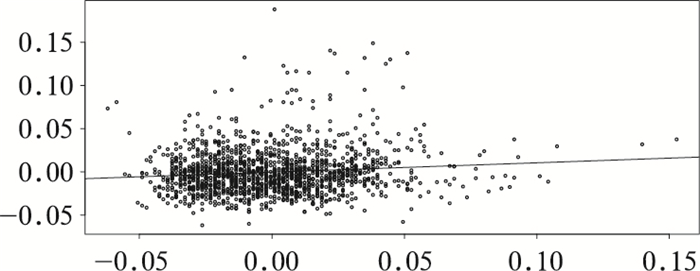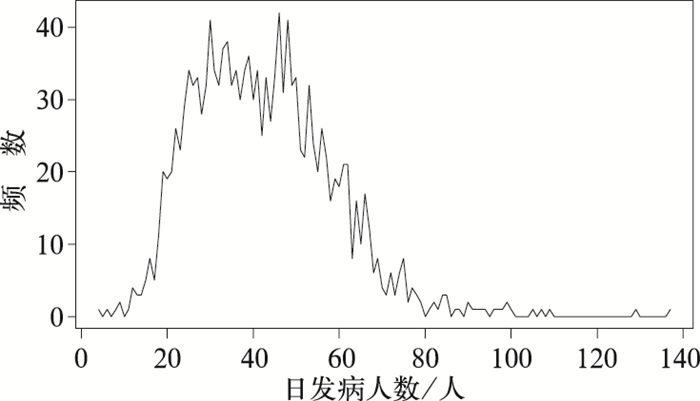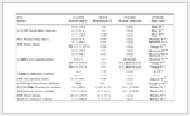﻿ 市域脑卒中疾病与气象因素的关系及预测
 快速检索 中文标题 英文标题 作者中文名 作者英文名 单位中文名 单位英文名 中文关键词 英文关键词 中文摘要 英文摘要 基金项目 全文
 气象2018, Vol. 44Issue (6): 837-843.  DOI: 10.7519/j.issn.1000-0526.2018.06.011### 引用本文 [复制中英文]

[复制中文]
CHENG Xuewei, HAN Zhaozhou, 2018. Relationship Between Stroke and Meteorological Factors and Its Prediction in Urban Areas[J]. Meteorological Monthly, 44(6): 837-843. DOI: 10.7519/j.issn.1000-0526.2018.06.011.
[复制英文]

### 文章历史

2017年9月15日收稿
2017年12月11日收修定稿

Relationship Between Stroke and Meteorological Factors and Its Prediction in Urban Areas
, HAN Zhaozhou
Department of Statistics, College of Economics, Jinan University, Guangzhou 510632
Abstract: The purpose of this paper is to help medical institutions in terms of a reasonable allocation of medical hands, beds and medical drugs, and also to help people in high stroke risk by mean of timely intervention, reducing the risk of stroke occurrence. The data of the cases of stroke in four hospitals from 2013 to 2016 were analyzed, and the number of daily patients in the hospital was divided into six grades. By taking the support vector machine (SVM) and the random forest (RF) methods, and using the corresponding local daily meteorological data collected, we established the prediction model for daily patients and the correlation model related to meteorological factors. The results showed that: (1) the number of daily patients with stroke was not balanced and the accuracy of traditional prediction model was relatively low. (2) By constantly adjusting the initial weight of the SVM prediction model, we found that, after 4 attempts of optimization, the prediction accuracy of the number of daily patients increased from 52.46% to 94.56%. (3) The results of the random forest model showed that the three meteorological factors affecting the incidence of stroke are the maximum temperature, the minimum temperature and the mean temperature. Thus, the research results of the correlation between stroke diseases and meteorological factors based on machine learning model could improve the prediction accuracy of medical meteorological statistical models, and have good application and promotion values.
Key words: stroke    meteorological factors    support vector mechine (SVM)    random forest (RF)    non-equilibrium data

1 资料与方法 1.1 资料来源

1.2 资料处理图 1 日就诊人数与天气因素的复相关系数图 Fig. 1 Complex correlation coefficient between the number of daily patients and weather factors图 2 脑卒中日就诊人数频数分布折线图 Fig. 2 Frequency distribution of the number of patients

1.3 支持向量机和随机森林方法 1.3.1 支持向量机方法

SVM最基础的原理就是将空间中的两类点(y=-1或y=1)用超平面wTx+b=0分开(在严格线性可分的情况下，存在这样的超平面)，而且希望这个超平面与两类的距离最大，也就是说，使得隔离带宽$\mathit{\rho }\text{=}\frac{2}{\left\| \mathit{w} \right\|}$最大。这等价于用Lagrange乘子法求下式的极小值。

 $\mathit{L}\left(\mathit{w}{\rm, }\mathit{b}{\rm, }\mathit{\alpha } \right){\rm =}\frac{1}{2}\|\mathit{w}{{\|}^{{\rm 2}}}{\rm -}\sum\limits_{\mathit{i}{\rm =1}}^{\mathit{n}}{{{\mathit{\alpha }}_{\mathit{i}}}} [ {{\mathit{y}}_{\mathit{i}}}{\rm (}{{\mathit{w}}^{\mathit{T}}}{{\mathit{x}}_{\mathit{i}}}{\rm +}\mathit{b}{\rm)-1 } ]$ (1)

 \begin{align} &\mathit{L}\left(\mathit{w}{\rm, }\mathit{b}{\rm, }\mathit{\xi }{\rm, }\mathit{\alpha }{\rm, }\mathit{\eta } \right){\rm =}\frac{{\rm 1}}{2}\|\mathit{w}{{\|}^{{\rm 2}}}{\rm +}\mathit{C}\sum\limits_{\mathit{i}}{{\rm (}}{{\mathit{\xi }}_{\mathit{i}}}{\rm +}\mathit{\xi }_{\mathit{i}}^{*}{\rm)-} \\ &\ \ \ \ \ \ \ \sum\limits_{\mathit{i}}{{\rm (}}{{\mathit{\eta }}_{\mathit{i}}}{{\mathit{\xi }}_{\mathit{i}}}{\rm +}\mathit{\eta }_{\mathit{i}}^{*}\mathit{\xi }_{\mathit{i}}^{*}{\rm)-}\sum\limits_{\mathit{i}}{{{\mathit{\alpha }}_{\mathit{i}}}}{\rm (}\mathit{\varepsilon }{\rm +}{{\mathit{\xi }}_{\mathit{i}}}{\rm -}{{\mathit{y}}_{\mathit{i}}}{\rm +} \\ &\ \ \ {{\mathit{w}}^{\mathit{T}}}{{\mathit{x}}_{\mathit{i}}}{\rm +}\mathit{b}{\rm)-}\sum\limits_{\mathit{i}}{\mathit{\alpha }_{\mathit{i}}^{*}}{\rm (}\mathit{\varepsilon }{\rm +}\mathit{\xi }_{\mathit{i}}^{*}{\rm +}{{\mathit{y}}_{\mathit{i}}}{\rm -}{{\mathit{w}}^{{\rm T}}}{{\mathit{x}}_{\mathit{i}}}{\rm -}\mathit{b}{\rm)} \\ \end{align} (2)

1.3.2 SVM针对不平衡数据的权重优化原理

1.3.3 随机森林方法

 $\mathit{Importance}{\rm =}\sum{{\rm (}}\mathit{errOO}{{\mathit{B}}_{{\rm 2}}}{\rm -}\mathit{errOO}{{\mathit{B}}_{{\rm 1}}}{\rm)/}\mathit{N}$ (3)

2 优化建模与日就诊人数等级预测表 1 脑卒中日就诊人数预报等级 Table 1 Grade prediction of the number of stroke patients

2.1 生成训练集和测试集

2.2 建立SVM模型及预测判别表 2 基于SVM的日就诊人数模型结果 Table 2 Results of daily number of patients based on SVM表 3 日就诊人数的预测结果的混淆矩阵 Table 3 Confusion matrix of predictions about the number of patients

2.3 优化SVM模型及预测判别表 4 4次优化对应的混淆矩阵 Table 4 Confusion matrix corresponding to four optimizations表 5 优化的分类权重与预测正确率 Table 5 Optimized classification weight and predictive accuracy

(1) 由表 3的混淆矩阵可知，前三类的特征变量不明显。需要调高模型前三类的权重，于是将分类权重从1：1：1：1：1：1优化为100：100：100：10：2：1。正确率从52.46%上升到了59.56%，且由表 4混淆矩阵①可得，日就诊人数等级为A1的，有7个预测正确，终于实现了A1与A2的特征区分。A3被预测正确的个数已由原来的2个上升为20个；

(2) 由混淆矩阵①可知，适当升高前三类的比重是有效果的，但是A1与A3中的错误数依旧远高于正确数。于是将比重从100：100：100：10：2：1优化为1000：1000：1000：100：2：1，这时的错误个数减少了32，正确率上升了近10%。但是A1与A3的错误数还是很高，证明SVM模型还是不能够很好地区分前三类的具体特征；

(3) 明确了提高前三类的比重对正确率有正向影响，于是将比重从1000：1000：1000：100：2：1优化为100000：100000：100000：1000：2：1，这次权重优化的幅度更大一点，前三类都提高了两个数量级。错误数减少了72，比上次优化的效果还好一点，正确率从68.31%上升为87.98%。从混淆矩阵③可知，此时的A1，A2，A3基本能够正确区分，并且B1的预测正确率也已经达到了50%；

(4) 从混淆矩阵④可知，虽然前三类的正确率不错，但是B2和B3的错误率100%，而且错的比较离谱。于是在第四次优化的过程中，增大前三类权重的同时，适当增大第四类、第五类的比重，将优化权重调为1000000：2000000：2000000：10000：2000：1，错误个数为20个，说明一年366 d中，仅有20 d预测错误，正确率达到了94.56%。并且除了B3这一组的预测差距比较大之外，其他组的错误基本保持在上下等级浮动。这一预测结果对于医疗机构合理调配医务力量、床位和医疗药物，具有现实性的指导意义。

3 探索影响日就诊人数的天气因素-建立随机森林模型表 6 影响日就诊人数的气象因素的重要性 Table 6 Importance of meteorological factors affecting the daily number of patients

4 结论

(1) 初期的数据挖掘发现，日就诊人数与8个气象因素呈现弱相关。但脑卒中的日就诊人数为不平衡数据，从折线图上反映出其为典型的右偏数据类型，这种数据特征是导致传统的预测模型预测脑卒中日就诊人数等级准确率较低的原因。

(2) 通过不断优化的SVM模型，使得日就诊人数等级的预测正确率达到94.56%，一年之中仅有20 d的日就诊人数等级预测错误。可以说，这种预测结果基本解决了医疗机构所面临的医务力量、床位和医疗药物的调配，具有一定的应用和推广价值。

(3) 最后，建立了日就诊人数关于气象变量的随机森林模型，探索出影响脑卒中发病率的三大重要气象因素：日最高气温、日最低气温、日平均气温。这将提醒脑卒中高危人群在温度变化异常的天气时，及时采取干预措施，降低发病风险。

 董平, 张敬华, 2000. 用圆形统计探讨老年心血管病发病的季节规律[J]. 数理统计与管理, 19(5): 31-33. 方万里, 马利娟, 2014. 宁波地区脑卒中发病规律与气象诱因统计分析[J]. 中国卫生统计, 31(1): 137-138. 黄衍, 查伟雄, 2012. 随机森林与支持向量机分类性能比较[J]. 软件, 33(6): 107-110. 梁丽英, 2009. 广州市气象因素和空气污染与脑卒中发病的相关性研究[D]. 广州: 广州医学院. http://www.wanfangdata.com.cn/details/detail.do?_type=degree&id=Y1554321 凌佳, 2013. 基于半参数回归模型的脑卒中发病率影响因素分析[J]. 数理医药学杂志, 26(1): 17-20. 吕红霞, 李志伟, 2013. 睡眠障碍与脑卒中的相关性研究进展[J]. 现代医药卫生, 29(17): 2620-2622. DOI:10.3969/j.issn.1009-5519.2013.17.028 闵晶晶, 丁德平, 李津, 等, 2014. 北京急性脑血管疾病与气象要素的关系及预测[J]. 气象, 40(1): 108-113. 阮烨, 2013. 冷空气活动对心脑血管疾病相关指标影响的初步研究[D]. 兰州: 兰州大学. http://cdmd.cnki.com.cn/Article/CDMD-10730-1014135079.htm 阮烨, 牛静萍, 张莉, 等, 2012. 冷空气活动对心脑血管疾病发生相关敏感指标影响的研究[J]. 环境与健康杂志, 29(6): 496-498. 阮烨, 牛静萍, 张莉, 等, 2013. 冷空气对心脑血管疾病患者血流变指标的影响[J]. 环境与健康杂志, 30(9): 773-776. 谭英, 耿德勤, 黄水平, 2013. 用人工神经网络建立缺血性脑卒中复发的预测模型[J]. 中国卫生统计, 30(5): 687-689. 王兴中, 祝锦涛, 1986. 急性脑血管疾病的发病与季节和异常气象的关系(合肥地区2142例分析)[J]. 安徽医学, 7(5): 1-3. 许显福, 裘建强, 1990. 急性脑卒中与气象要素的探讨[J]. 浙江气象科技, 11(1): 33-35. 杨贤为, 邹旭恺, 1998. 北京地区脑卒中发病率的气象条件研究[J]. 气象, 24(9): 51-54. 印佩芳, 马辛宇, 袁军, 等, 1993. 脑卒中与天气过程的关系[J]. 气象, 19(12): 44-47, 53. 张书余, 牛静萍, 罗斌, 等, 2013. 冷空气对心脑血管疾病的影响及其机制研究[M]. 北京: 气象出版社. 张书余, 王宝鉴, 谢静芳, 等, 2010. 吉林省心脑血管疾病与气象条件关系分析和预报研究[J]. 气象, 36(9): 106-110. 张书余, 张夏琨, 崔世杰, 等, 2016. 冷空气对人心血管系统及相关影响因素的自然实验研究[J]. 气象, 42(10): 1256-1262. 赵娜娟, 牛静萍, 阮烨, 等, 2011. 冷空气活动对人群血脂水平的影响[J]. 环境与健康杂志, 28(11): 953-955. Chawla N V, Bowyer K W, Hall L O, et al, 2002. SMOTE:synthetic minority over-sampling technique[J]. J Artif Intell Res, 16(1): 321-357. Cowperthwaite M C, Burnett M G, 2011. An analysis of admissions from 155 United States hospitals to determine the influence of weather on stroke incidence[J]. J Clin Neurosci, 18(5): 618-623. DOI:10.1016/j.jocn.2010.08.035 Wang Qinzhou, Gao Cuilian, Liu Hongen, et al, 2017. Hypertension modifies the short-term effects of temperature on morbidity of hemorrhagic stroke[J]. Sci Total Environ, 598: 198-203. DOI:10.1016/j.scitotenv.2017.04.159